# Computational project part 1

We will use the Euler method to solve the damped pendulum equation written as a systemfor initial conditions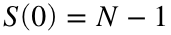,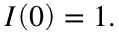I'm going to assume the disease is spreading on a small island with a population of 10000 people and that it starts when a single infected person arrives on the island..
% Set up initial conditions and parameters
N = 10000;
S0=N-1;
I0=1;
beta=.0002;
gamma=1;
% Set up time stepping
h = 1/100;
tfinal=20;
nsteps=tfinal/h;
t=linspace(0,tfinal,nsteps+1);
% Initialize x & y
S=zeros(size(t));
I = zeros(size(t));
S(1)=S0; I(1)=I0;
% Loop through and run the Euler method
for k=1:nsteps
S(k+1) = S(k) + h * -beta*S(k)*I(k);
I(k+1) = I(k) + h * (beta*S(k)-gamma)*I(k);
end
% plot the results
plot(t,S,t,I);
xlabel('t'); legend('S','I')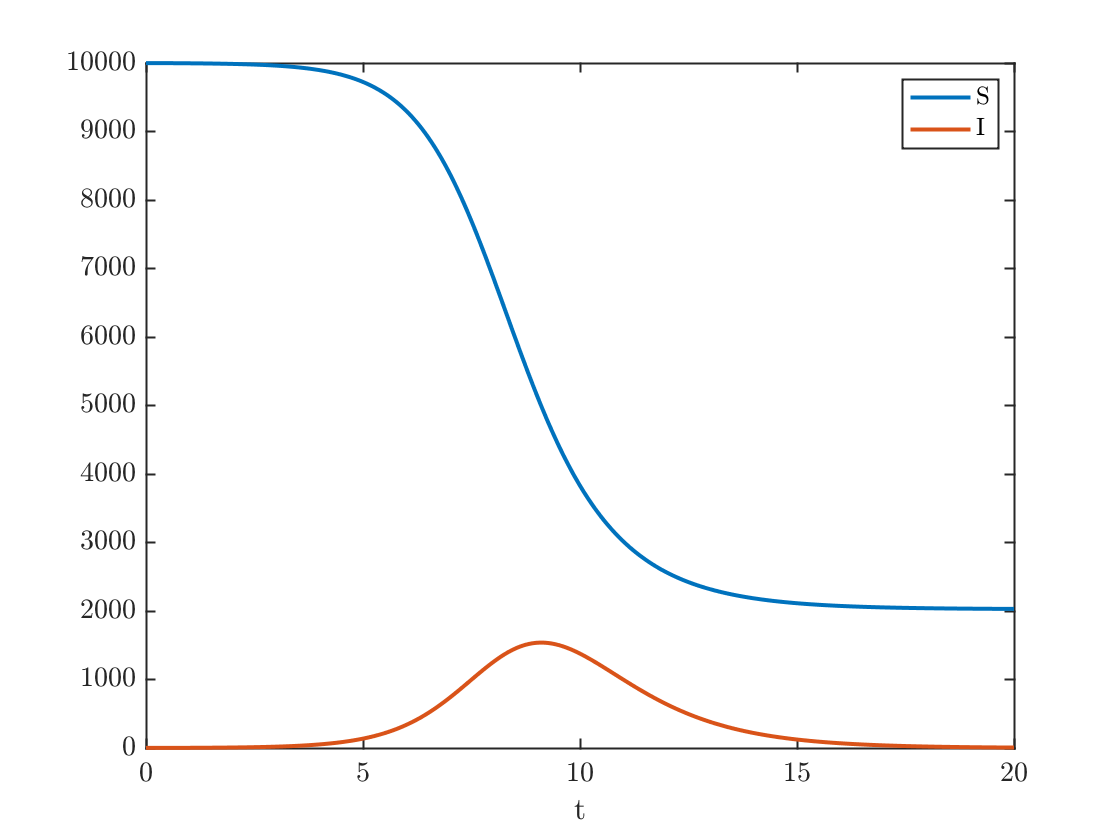## Plot the solution in the phase plane

plot(S,I,S(1),I(1),'o')
xlabel('S');ylabel('I');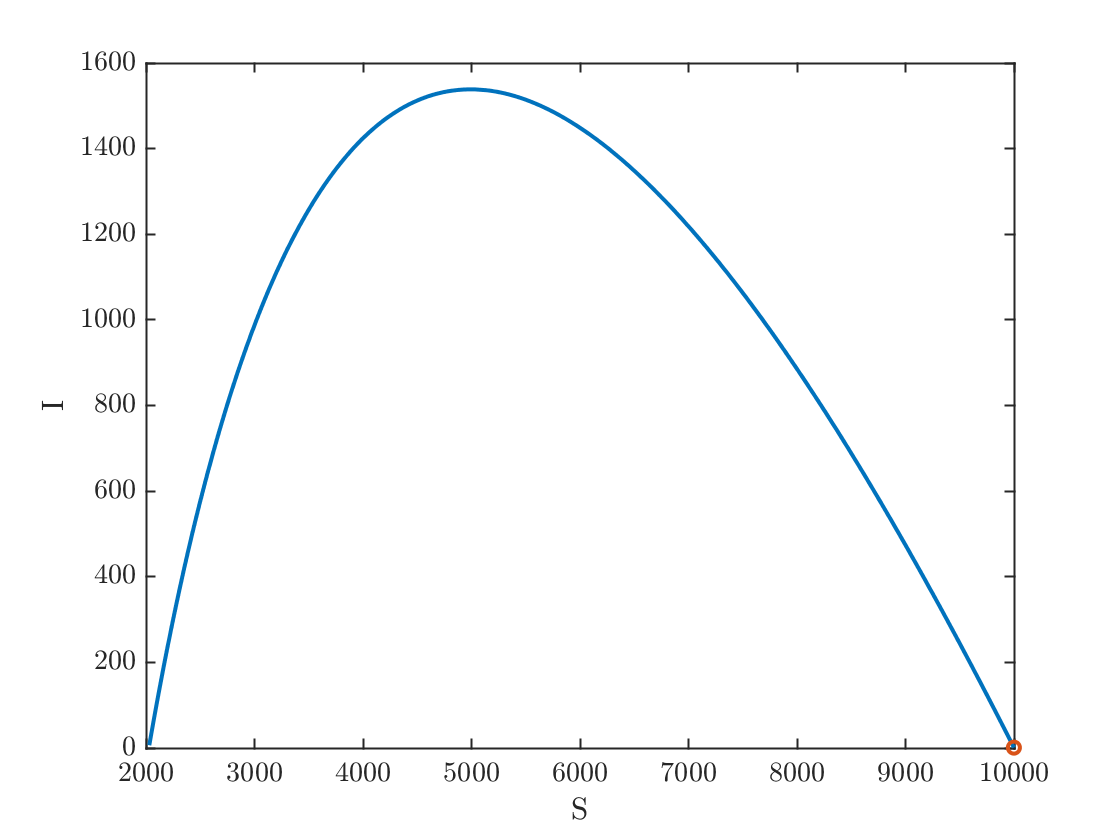fprintf('%0.1f%% of the population eventually caught the disease.\n',100*(N-S(end))/N)
79.7% of the population eventually caught the disease.
fprintf('At the epidemic''s peak, %0.1f%% of the population was infected.\n',100*max(I)/N)
At the epidemic's peak, 15.4% of the population was infected.

#### Now, suppose the government had implemented a stay-at-home order, which causes β to decrease to a avalue of 0.00125.

The epidemic now proceeds more slowly, so we have to run the simulation longer. We see that the total number of infected people, and the peak of the outbreak are significantly reduced.
beta=.000125;
tfinal=50;
nsteps=tfinal/h;
t=linspace(0,tfinal,nsteps+1);
% Initialize x & y
S=zeros(size(t));
I = zeros(size(t));
S(1)=S0; I(1)=I0;
% Loop through and run the Euler method
for k=1:nsteps
S(k+1) = S(k) + h * -beta*S(k)*I(k);
I(k+1) = I(k) + h * (beta*S(k)-gamma)*I(k);
end
% plot the results
plot(t,S,t,I);
xlabel('t'); legend('S','I')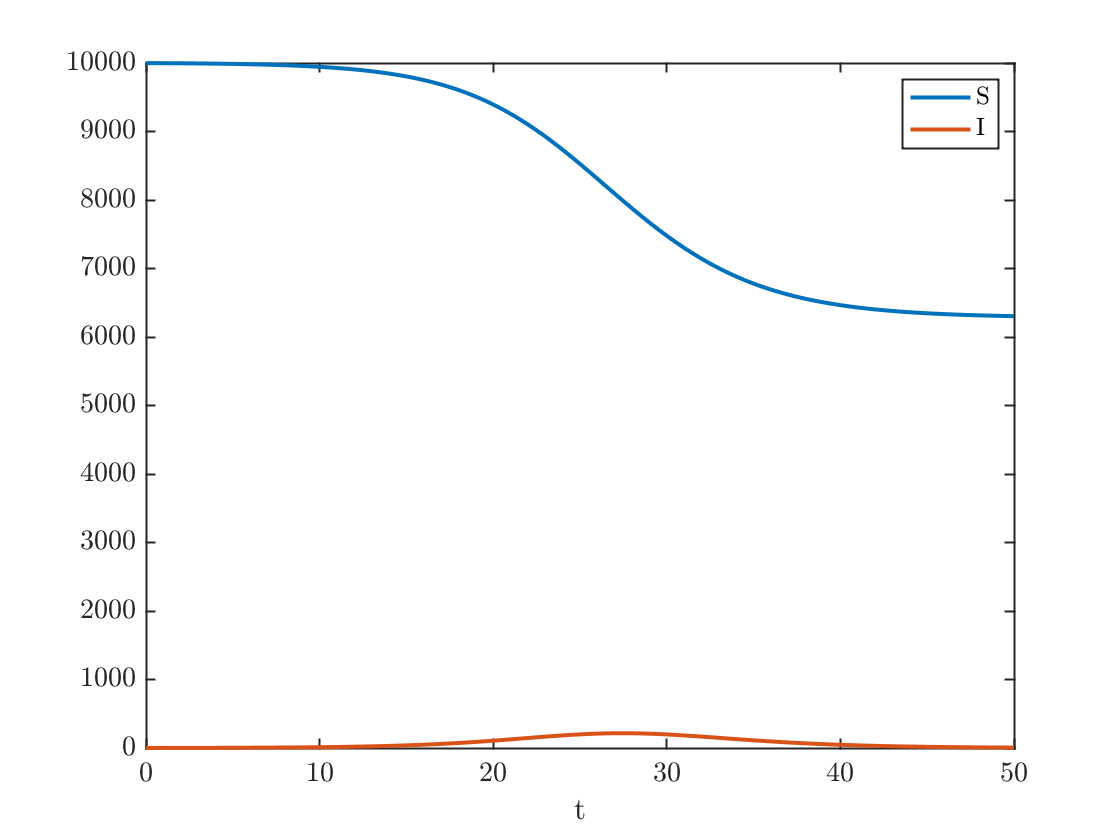#### Plot the solution in the phase plane

plot(S,I,S(1),I(1),'o')
xlabel('S');ylabel('I');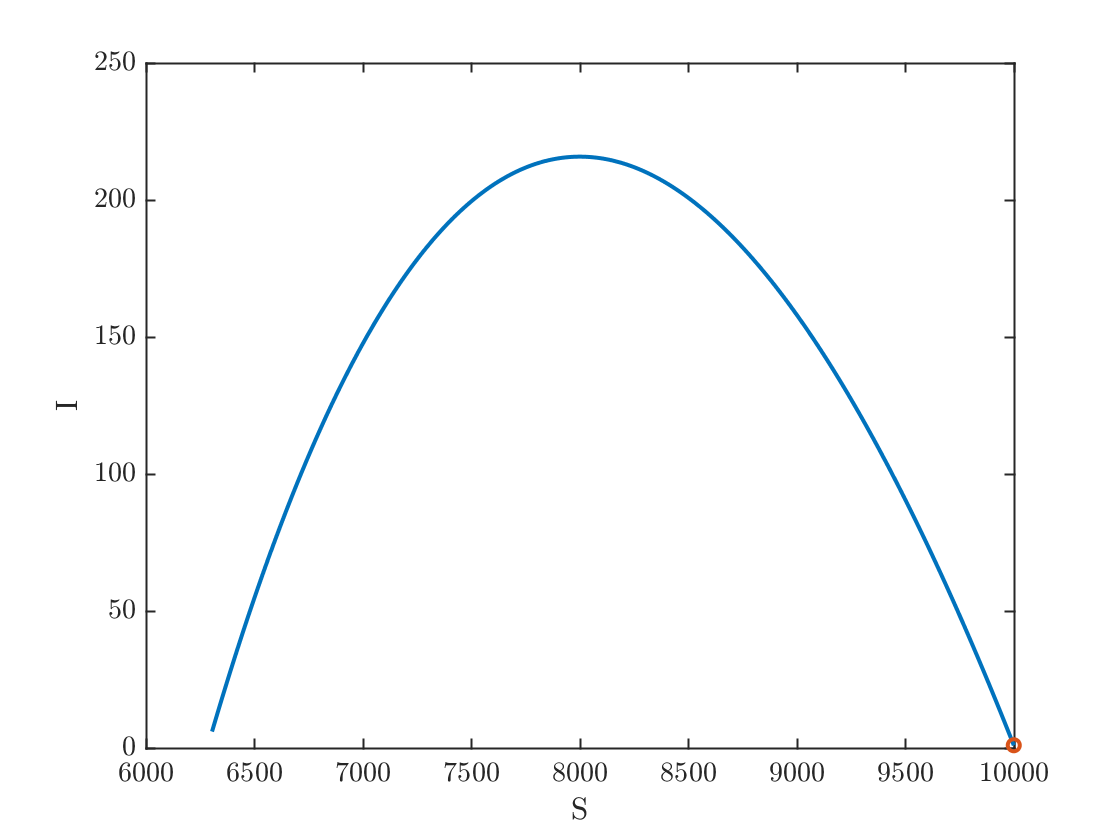fprintf('%0.1f%% of the population eventually caught the disease.\n',100*(N-S(end))/N)
37.0% of the population eventually caught the disease.
fprintf('At the epidemic''s peak, %0.1f%% of the population was infected.\n',100*max(I)/N)
At the epidemic's peak, 2.2% of the population was infected.

#### Now, suppose the government had implemented a stay-at-home order, and people are disciplined about social-distancing and mask wearing, which causes β to decrease to a avalue of 0.0009.

In this case,and the pandemic never takes off. As a result, only 10 people ever get sick.
beta=.00009;
tfinal=50;
nsteps=tfinal/h;
t=linspace(0,tfinal,nsteps+1);
% Initialize x & y
S=zeros(size(t));
I = zeros(size(t));
S(1)=S0; I(1)=I0;
% Loop through and run the Euler method
for k=1:nsteps
S(k+1) = S(k) + h * -beta*S(k)*I(k);
I(k+1) = I(k) + h * (beta*S(k)-gamma)*I(k);
end
% plot the results
plot(t,S,t,I);
xlabel('t'); legend('S','I')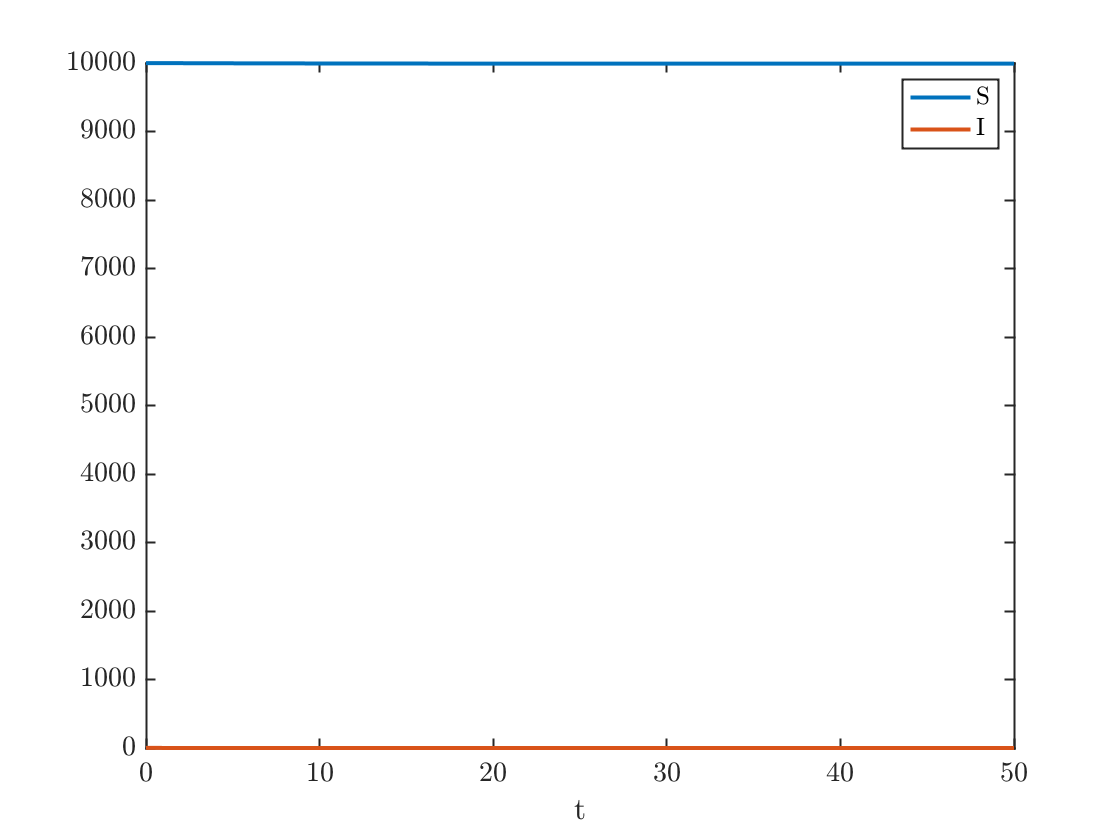## Plot the solution in the phase plane

plot(S,I,S(1),I(1),'o')
xlabel('S');ylabel('I');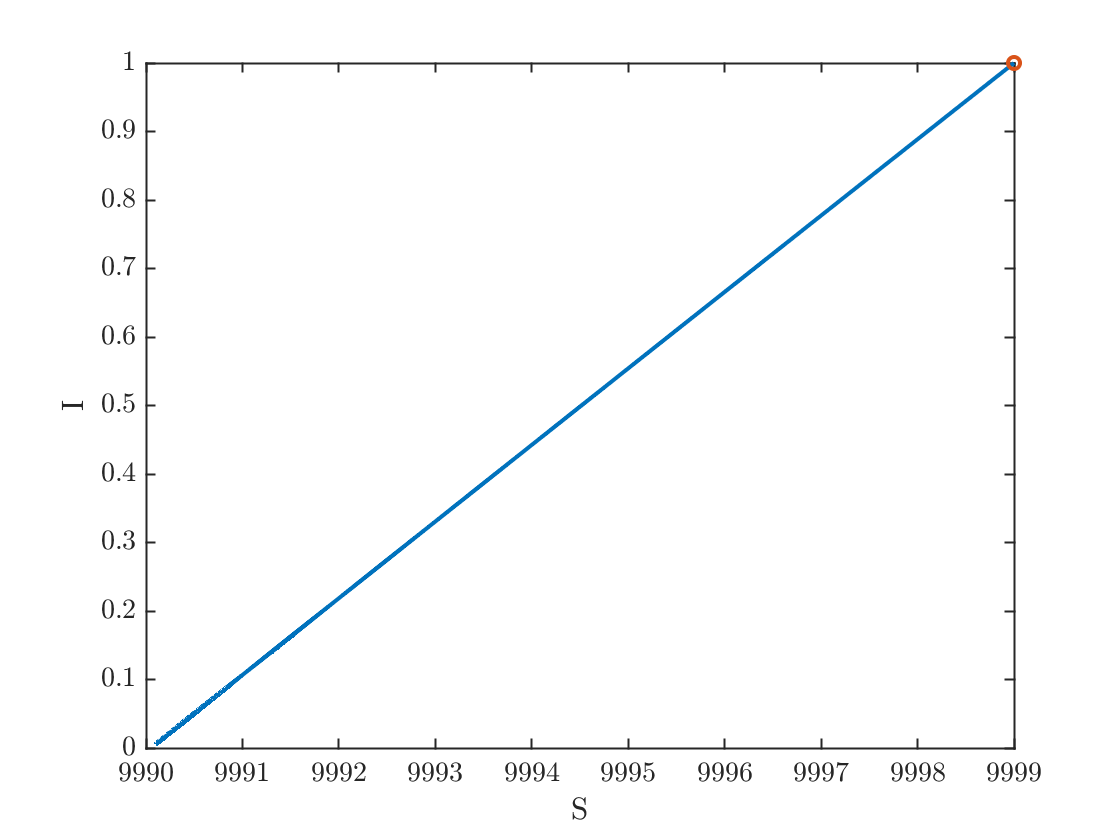fprintf('%0.1f%% of the population eventually caught the disease.\n',100*(N-S(end))/N)
0.1% of the population eventually caught the disease.
fprintf('At the epidemic''s peak, %0.1f%% of the population was infected.\n',100*max(I)/N)
At the epidemic's peak, 0.0% of the population was infected.